## General Question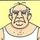# How do you measure the number of acres in a section?

Asked by srmorgan (6768) March 8th, 2008

I am having trouble conceptualizing this and yes I have looked at Google! If a section is one square mile with 640 acres, then how many acres is it lengthwise and how many acres is it widthwise? I just can’t take this from an abstraction to a physical representation.

Observing members: 0Composing members: 0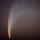An acre is a unit of area not of length, so I can see why you are having trouble conceptualizing it.

tekn0lust (1861)“Great Answer” (0) Flag as…ok
A section is 640 acres or one square mile. So imagine if we divide the section into quadrants. The first quadrant is now 160 acres or one-quarter square mile, so this segment is 2,640 feet by 2,640 feet, is that right?
So I guess my problem is that while you can have 640 acres in a square mile, those 640 acres can not be configured into the square mile AS SQUARES. Is that right?

Or the proverbial “40 acres and a mule” was a section of land 1/4 mile by 1/4 mile, or 1,742,700 square feet, but each acre would be a rectangle and not a perfect square?

SRM

srmorgan (6768)“Great Answer” (0) Flag as…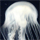1/4 of a mile by 1/4 of a mile is 160 acres, not 40.

sqrt(40) is not a whole number, so no matter how hard you try, you cannot lay out 40 squares in a square.

the same area of 40 acres is in a 1/8 mile bye 1/8 mile patch, though.

aaronblohowiak (485)“Great Answer” (1) Flag as…Thanks, it was the fact that you couldn’t lay out the acres, whether 40, 160 or 640 into squares that was throwing me.
SRM

srmorgan (6768)“Great Answer” (0) Flag as…srmorgan (6768)“Great Answer” (0) Flag as…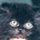hey aaron—do the math or read the question before you answer. srmorgan said “one-quarter square mile”, not one-quarter mile square. so, this person was correct when they said one-quarter square mile is 160 acres. (1/2×1/2 = 1/4—this is basic math—do you have a problem with fractions?) and, as you noted, there is square root of 40 (approx 6.324) so you can have 40 acres in a square. last—1/4 mile by 1/4 mile IS 40 acres, not the 160 you said it is. again—fractions: 1/4×1/4 = 1/16 or 640 acres divided by 16 = 40.

pololo (1)“Great Answer” (0) Flag as…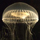Each side is approx. 25.3 acres long. If my caculations are correct.Or each side of a square acre is 208.71 feet so that would total 5280,363 feet long on each side. I humbly submit this as my answer. If I’m wrong (which in this case i do not think that I am) I hope that someone can tell me where I may have gone wrong.
Thanks trevorc5733

trevorc5733 (1)“Great Answer” (0) Flag as…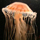First off the sections and township measurements are approximate. Because of the curvature of the earth you would need calculus to figure the measurements and still they would be only approximation just more accurate. An acre is a measure in SQUARE FEET; therefore you cant not measure in square acres. An acre can be square but rarely is. Acres are to conceptualize large numbers. there are 27,878,400 square feet in a section. That number is meaningless due to the conceptualization problem. However when you say there 640 acres in a section that number is more easy to grasp. You can not have the square root of acre ie the square root of 40 acres is a meaningless term. 40 acres is 43,560 square feet x 40 = 1.742,400 square ft. take the square root = 1320. Therefore 40 acres is 1320 ft on each side. trev’s answer is essentially correct, tho his phrasing is confusing. You would take 5280 ft the side of a section (because this is an absolute known quantity) divide by the 208.71 figure to get the 25.298 square acres on a side of a section. If that helps great but otherwise it is not a very useful number. Finally land was formerly commonly sold buy section, ½ section, ¼ section etc. you would see a legal description like the NW (northwest) quarter of section so and so. or the N half of that section. or the east half of the north half of the northwest quart of section so and so. then you have begining at 80 ft south of the northwest corner of the east half of section so and so and proceeding west 240 feet thence north 400 feet thence to the point of beginning. That describes a triangle. fortunately in our town they went to subdivision and that makes things a lot simpler.

billdavis (6)“Great Answer” (0) Flag as…Yes, you can divide that area into squares – just not 640 squares of exactly one acre each – if the original square mile plot is a square. And, if your square mile plot is NOT square (for example, imaging a rectangle 64 square acres long and 10 square acres wide) – you can. Assuming the plot has to be a square – conceptualize this… Image 5280 squares across and 5280 squares down – each square with 1 foot edges. OK? Now, we will combine four adjacent squares – imagine 2640 squares across and 2640 squares down – each square with 2 foot edges. 1320×1320 squares with 4’ edges. 660×660 with 8’ edges. 330×330 with 16’ edges. 165×165 with 32’ edges. If we now combine 5×5 squares into one, you get 33×33 squares each with 160’ edges.

frankfazzio (1)“Great Answer” (0) Flag as…or